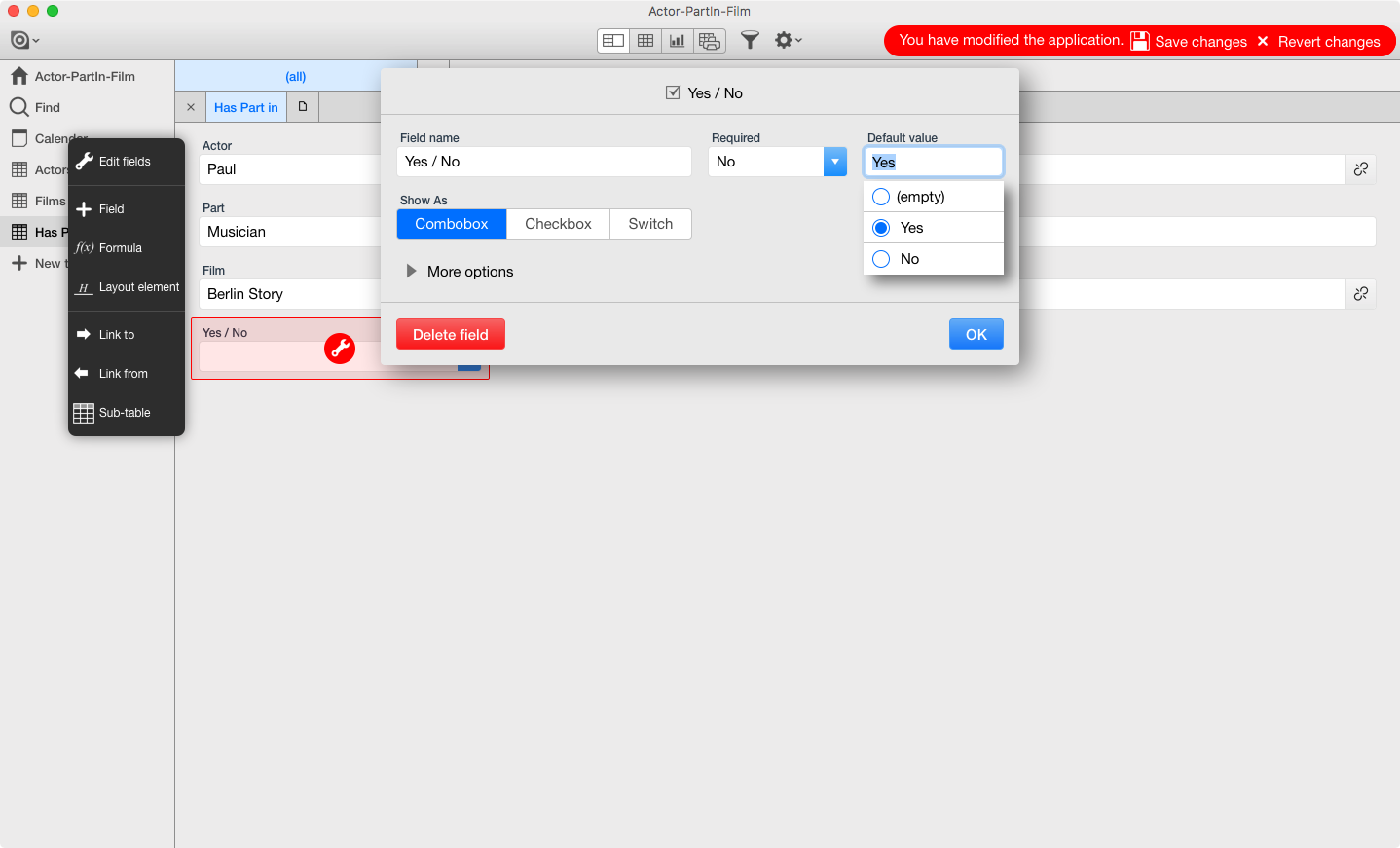Love the ease of this data base software, but im having trouble trying to work out a formula to do a task I would like on a database which is:

IF"SOLD" = "YES" (from a yes/no choice field) then "VALUE \$ = 0" in a value field . ie. trying to get a data field to show 0\$ if the sold field = Yes, or alternatively if a "sold date" field has a date in it then \$0 in the Value field.

Hope this makes sense!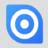If you like to compare text like „YES“ or „NO“ with the shown text in a choice field, you have to do as follows: Either you use the index from your Choice values list: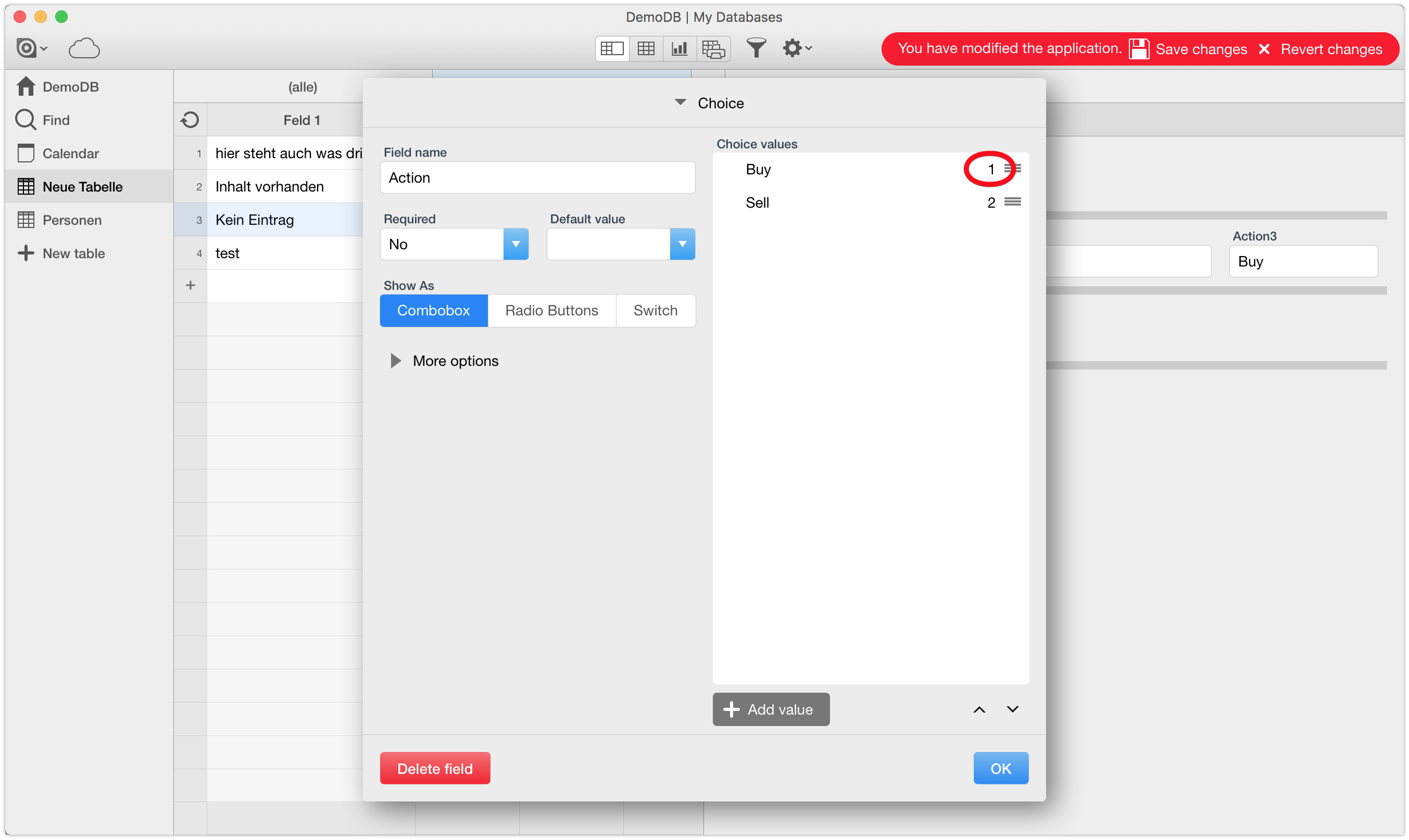Formula:

if SOLD = 1 then "VALUE \$ = 0"

or you have to declare that you want to compare the text in the SOLD field. Then the formula has to look like that:

if text(SOLD) = "YES" then "VALUE \$ = 0"

Kind regards, Jörg

Brilliant, thanks Jörg, just what I wanted. Works perfectly.

Ah....but I just created another (unforseen) design issue. Is it possible to have 2 agruments in a cell? ..or is this now getting too compicated :)

1. if text(SOLD) = "YES" then "VALUE \$ = 0"

Plus:

2. if text(SOLD) = "NO" then "VALUE \$ = (remains is the same as it is in "COST" field)" ??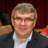Hi Ray,

"Yes / No" field has three options:
Yes (true)
No (false)
Empty (null).
You can configure the "after change" trigger like this:

'VALUE \$':= if SOLD=true then 0 else COST

regards

Leo

Hi How to remove the (empty) option from choice menu ?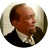Give the field an inital (default) value: